### Home > CAAC > Chapter 4 > Lesson 4.2.4 > Problem4-98

4-98.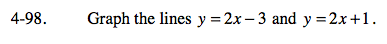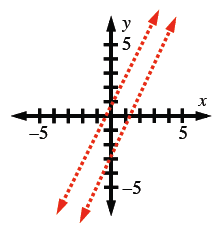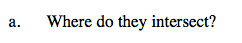Do parallel lines ever intersect?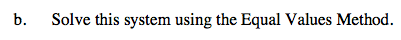Set the equations equal to each other.

y = y
2x − 3 = 2x + 1

Subtract 2x from both sides. What happens?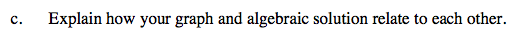What do the two methods have in common?